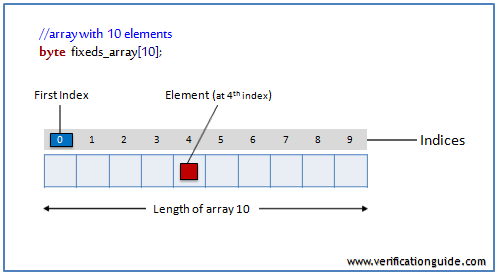# Array in SystemVerilog

## Array

An array is a collection of variables, all of the same type, and accessed using the same name plus one or more indices. there are different types of arrays, few array declaration examples are given below.

```int array1 ;            //fixed size single dimension array
int array2 [5:0];          //fixed size single dimension array
int array3 [3:0][2:0];     //fixed size multi dimension array
bit [7:0] array4[2:0];     //unpacked array declaration
bit [2:0][7:0] array5;     //packed array declaration
bit [2:0][7:0] array6 ; //mixed packed and unpacked array
```fixed size array in SystemVerilog

In SystemVerilog vector width/dimensions declared before the object name is referred to as packed array and array size/dimensions declared after the object name is referred to as an unpacked array.

A packed array is a mechanism for subdividing a vector into sub-fields which can be conveniently accessed as array elements.

## Fixed size array

In fixed size array, array size will be constant throughout the simulation, Once the array is declared no need to create it. By default, the array will be initialized with value ‘0’.

### Single dimensional array

```int array1 ;   //Compact declaration
int array2 [5:0]; // Verbose declaration
```

### Multidimensional array

Multidimensional arrays are also known as an array of arrays.

#### Two-dimensional array

```int arr;
```

This array has total 2*3 = 6 elements.

#### Three-dimensional array

```int arr;
```

This array has total 2*2*2 = 8 elements.

#### Two-dimensional array declaration

```int array3 [2:0][3:0];
```

The data in a two-dimensional array is stored in a tabular form as shown in the below diagram.SystemVerilog multidimensional array

## array assignment

```array1 = '{0,1,2,3,4,5};
array2 = '{0,1,2,3,4,5};
array3 = '{ '{0,1,2,3},'{4,5,6,7},'{8,9,10,11}};
```

### Fixed Size Array Example

This example shows array declaration and array manipulation using for and foreach loop.

```module fixedsize_array;
//declaration of array’s
int array1;               //single dimension array
int array2[5:0];             //single dimension array
int array3[2:0][3:0];        //multi dimension array
int array4[4:0];

initial begin
//array initialization
array1 = '{0,1,2,3,4,5};
array2 = '{0,1,2,3,4,5};
array3 = '{'{0,1,2,3},'{4,5,6,7},'{8,9,10,11}};

//displaying array elements
\$display("-------displaying array1-------");
foreach(array1[i]) \$display("\t array1[%0d] = %0d",i,array1[i]);

\$display("-------displaying array2-------");
for(int i=0;i<6;i++) \$display("\t array2[%0d] = %0d",i,array2[i]);

\$display("-------displaying array3-------");
foreach(array3[i,j]) \$display("\t array3[%0d][%0d] = %0d",i,j,array3[i][j]);

\$display("-------displaying uninitialized array4-------");
for(int i=0;i<5;i++) \$display("\t array4[%0d] = %0d",i,array4[i]);
end
endmodule```

Simulator Output

-------displaying array1-------array1 = 0
array1 = 1
array1 = 2
array1 = 3
array1 = 4
array1 = 5
-------displaying array2-------
array2 = 5
array2 = 4
array2 = 3
array2 = 2
array2 = 1
array2 = 0
-------displaying array3-------
array3 = 0
array3 = 1
array3 = 2
array3 = 3
array3 = 4
array3 = 5
array3 = 6
array3 = 7
array3 = 8
array3 = 9
array3 = 10
array3 = 11
-------displaying uninitialized array4-------
array4 = 0
array4 = 0
array4 = 0
array4 = 0
array4 = 0